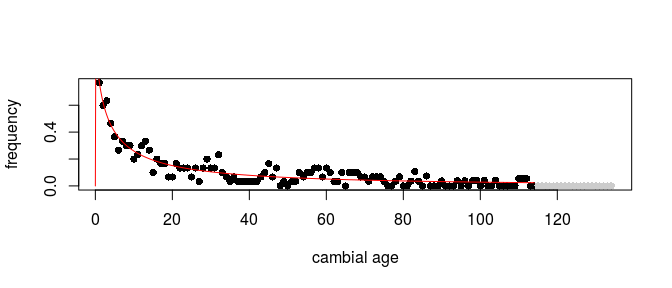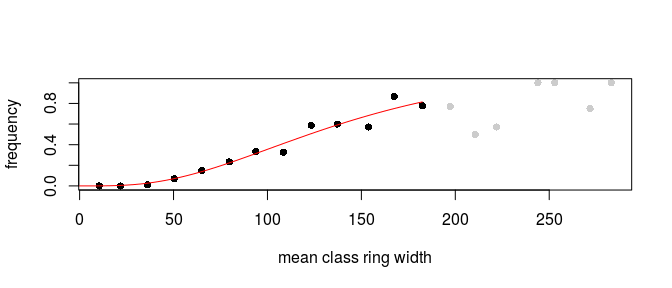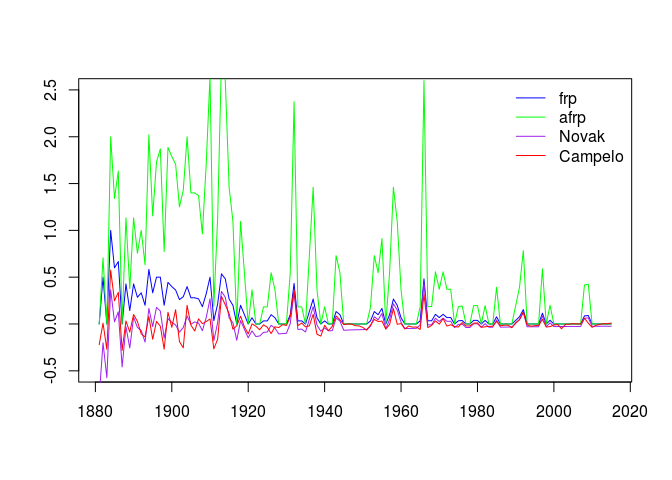# Introduction

Intra annual density fluctuations (iadfs), also referred as false rings are defined as either latewood-like cells in earlywood or earlywood-like cells in latewood (Fritts 1976). Although recent efforts are made to specify the position, extend as well as intensity of iadfs most studies use binary assignments to indicate presence or absence of iadfs (Battipaglia et al. 2016). While frequencies can easily be calculated as the proportion of rings showing an iadf in a specific year several studies suggest the consideration of variing variances, age trends as well as influence of ring size. This package implements all published approaches known by the package author, their usage is shortly explained in this vignette.

# Data

The data used in this vignette is included in the package. You can load the data-sets called example_rwl and example_iadf by typing:

library("iadf")
data("example_rwl")
data("example_iadf")

example_rwl is a data frame, similar to the class rwl defined by the package dplR with series as columns and years as rows, rownames specifying the year. The data shows the ring width with years not covered by the sample marked with NA.

BawPN05b BawPN06a BawPN06b BawPN07a BawPN07b BawPN08a BawPN08b
1900 NA NA NA NA NA 246 226
1901 NA NA NA NA NA 184 156
1902 209 NA NA NA NA 208 166
1903 265 NA 255 NA NA 213 178
1904 114 113 105 NA NA 116 90
1905 160 141 121 NA NA 132 115
1906 170 124 248 NA NA 158 156
1907 112 75 188 188 NA 123 71
1908 93 73 115 179 178 91 50
1909 135 80 116 225 215 127 89
1910 151 117 137 290 219 145 120

example_iadf has the same dimensions as example_rwl, just showing binary assignements of iadfs instead of ring width.

BawPN05b BawPN06a BawPN06b BawPN07a BawPN07b BawPN08a BawPN08b
1900 NA NA NA NA NA 1 1
1901 NA NA NA NA NA 1 1
1902 1 NA NA NA NA 0 1
1903 0 NA 1 NA NA 0 0
1904 1 1 0 NA NA 0 0
1905 1 1 1 NA NA 1 0
1906 0 1 1 NA NA 1 0
1907 0 0 0 1 NA 1 0
1908 0 0 0 1 1 0 0
1909 0 0 0 1 1 1 0
1910 1 1 1 1 1 1 0

# False-ring proportions

## Naive approach

The proportion of rings showing an iadf can easily be calculated using base R:

rowMeans(example_iadf, na.rm = TRUE)

However, there’s also a function in the package iadf to calculate the false ring proportion with data frame output consistent to the other package functions and improved warning messages.

results_frp <- frp(example_iadf)

Please mention that this function is somehow slower than using rowMeans(), so consider using the base R code whenever computation speed is critical.

## Consideration of sample size

As the variance of time series is dependend on sample size the variance can be adjusted according to Osborn (1997), using the function afrp():

results_afrp <- afrp(example_iadf)

## Consideration of age trend

As other tree ring parameters, also IADF occurence shows an age trend. Novak (2013) suggested a detrending procedure to reduce this bias.

First the iadf frequency per cambial age needs to be calculated:

frq <- novak_freq(example_iadf)

Then we try to model the influence of age on iadf frequency using a Weibull function as suggested by Novak (2013), limiting the data pairs used to cambial ages representing at least 15 years:

mdl <- novak_weibull(frq, 15)If you encounter an error its likely due to insufficient starting values for the curve fitting function (which can be found using novak_weibull_find_start() and will be discussed for campelo_chapman_find_start()in the next section).

Next we hand the model and the original data to the function novak_index() to calculate the iadf proportion with age trend removed:

results_novak <- novak_index(example_iadf, mdl)

## Consideration of the influence of ring width

Campelo (2014) states that beneath ring age also ring width influences iadf formation and introduced another standardization approach.

The workflow implemented in iadf is almost the same as for the approach above. Fist we calculate frequencies per ring width class using both data sets:

frq <- campelo_freq(example_iadf, example_rwl)

Then we fit a chapman function to our frequencies:

mdl <- campelo_chapman(frq)In case the function throws an error it’s likely due to insufficient starting values. These can be found interactively with campelo_chapman_find_start() and then used in campelo_chapman():

st <- campelo_chapman_find_start(frq)
mdl <- campelo_chapman(frq, start = st)

Next the index is calculated using both data sets and the model:

results_campelo <- campelo_index(example_iadf, example_rwl, mdl)

# Comparison of the approaches

plot(NULL, xlim = range(as.numeric(rownames(example_iadf))),
ylim = c(-0.5, 2.5), xlab = '', ylab = '')
lines(results_frp, col = 'blue')
lines(results_afrp, col = 'green')
lines(results_novak, col = 'purple')
lines(results_campelo[ , c(1,3)], col = 'red')
legend('topright', col = c('blue', 'green', 'purple', 'red'),
legend = c('frp', 'afrp', 'Novak', 'Campelo'), bty = 'n', lty = 1)